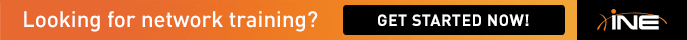# FR0 switch to R5 problem

Hi all,

I am using ine.routing.and.switching.topology.5.00-AG.gns3 - working 29.9.11.net file which came with Vol2 Dynamips edition workbook. Have you anybody encountered problem between Frame-relay switch and R5 S0/0 interface? The serial interface is UP but not receiving any LMIs. I tried all different lmi-types but can't get it working.

Any help would be appreciated.

The relevant configs are below

[[ROUTER R5]]

model = 3725

console = 2005

aux = 2206

cnfg = initial.configs/R5.cfg

wic0/0 = WIC-1T

wic0/1 = WIC-1T

wic0/2 = WIC-1T

s0/0 = FR0 6

s0/1 = R4 s0/1

f0/0 = SW1 f1/5

f0/1 = SW3 f1/5

x = -501.589066076

y = 256.769229133

hx = 22.4734458636

hy = 44.3136388535

[[FRSW FR0]]

1:102 = 2:201

1:103 = 3:301

1:104 = 5:401

1:105 = 6:501

1:113 = 4:311

2:201 = 1:102

2:203 = 3:302

2:204 = 5:402

2:205 = 6:502

2:213 = 4:312

3:301 = 1:103

3:302 = 2:203

3:304 = 5:403

3:305 = 6:503

4:311 = 1:113

4:312 = 2:213

4:314 = 5:413

4:315 = 6:513

5:401 = 1:104

5:402 = 2:204

5:403 = 3:304

5:405 = 6:504

5:413 = 4:314

6:501 = 1:105

6:502 = 2:205

6:503 = 3:305

6:504 = 5:405

6:513 = 4:315

1 = R1 s0/0

2 = R2 s0/0

3 = R3 s1/0

4 = R3 s1/1

5 = R4 s0/0

6 = R5 s0/0

x = -469.5

y = 77.5